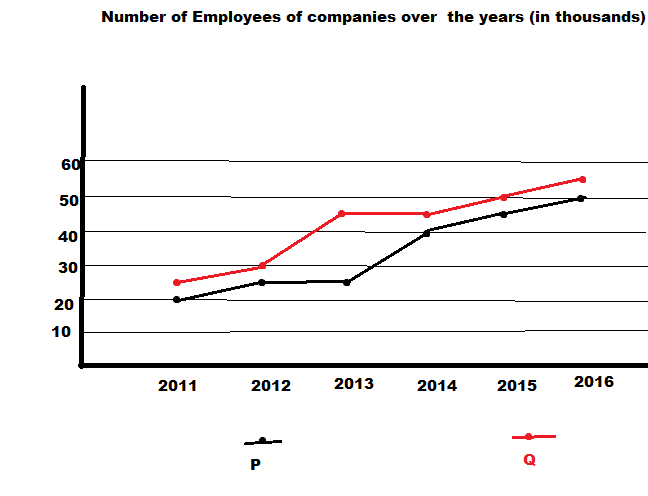QuestionAnswers

# What is the approximate percentage increase in the total employees of the companies from 2013 to 2016?A.50%B.10.5%C.9.5%D.9.2%

Hint: From the graph , first calculate the total number of employees in both the companies act 2013 and the total number of employees in both the companies at 2016. Then calculate the percentage increase using the formula $\dfrac{{increase{\text{ }}in{\text{ }}number}}{{{\text{original value}}}}*100$

Step 1 :
From the graph ,
The number of employees at company P in the year 2013 is 25000
The number of employees at company Q in the year 2013 is 45000
Therefore the total number of workers in both the companies act 2013 = $25000 + 45000 = 70000$
Step 2:
Now from the graph,
The number of employees at company P in the year 2016 is 50000
The number of employees at company Q in the year 2016 is 55000
Therefore the total number of workers in both the companies at 2016 = $50000 + 55000 = 105000$
Step 3 :
Now we are asked to find the percentage increase of total employees of both the companies.
Increase in percentage =$\dfrac{{increase{\text{ }}in{\text{ }}number}}{{{\text{original value}}}}*100$
Here the increase in number is the difference between the number of employees in both the companies at 2016 and the total number of employees of both the companies act 2013.
And the original value is the number of employees in 2013.
Therefore ,
Percentage increase
$= \dfrac{{105000 - 70000}}{{70000}}*100 \\ = \dfrac{{35000}}{{70000}}*100 \\ = \dfrac{1}{2}*100 \\ = 50\% \\$
Therefore the increase in percentage of the total number of employees in both the companies is 50%

The correct option is A.

Note: Steps used to calculate the percentage increase:
1. Calculate the difference (increase) between the two numbers you are comparing.
2.Increase = New Number - Original Number.
3. Divide the increase by the original number and multiply the answer by 100.
4.% increase = Increase ÷ Original Number × 100.